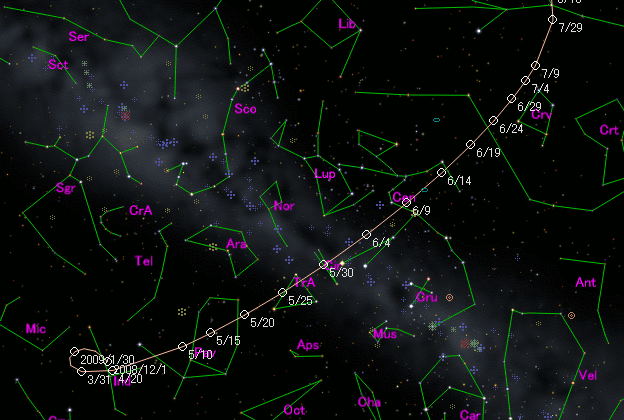# \$B%.%c%i%C%IWB@1(B###\$B%W%m%U%#!<%k(B

 \$BH/8+F|(B 2008\$BG/(B8\$B7n(B27\$BF|(B \$BH/8+8wEY(B 19.2\$BEy(B \$BH/8+ G. J. Garradd (Siding Spring Survey)

###\$B###\$B50F;MWAG(B

```Epoch 2009 June 18.0 TT = JDT 2455000.5
T 2009 June 23.08980 TT                                 MPC
q   1.7982217            (2000.0)            P               Q
z  +0.0001425      Peri.  340.85360     -0.56422670     -0.71956117
+/-0.0000007      Node   219.73407     -0.65050452     +0.08550228
e   0.9997438      Incl.  140.70604     -0.50842118     +0.68914518
From 624 observations 2009 May 3-2010 June 3, mean residual 0".6.
```

###\$B@1?^(B###\$B8wEYJQ2=(B

```        m1 = -0.3 + 5 log\$B&\$(B + 28.5 log r  [   ,130]  (              \$B!A(B2009\$BG/(B10\$B7n(B31\$BF|(B)
m1 =  3.0 + 5 log\$B&\$(B + 20.0 log r  [130,   ]  (2009\$BG/(B10\$B7n(B31\$BF|!A(B              )
```##### \$B50F;MWAG\$O(BM.P.E.C. 2012-U109\$B\$K7G:\\$5\$l\$?\$b\$N\$G\$9!#(B \$B@1?^\$O%9%F%i%J%S%2!<%?(B Ver.8 (\$B%"%9%H%m%"!<%D(B) \$B\$G:n@.\$7\$?\$b\$N\$G\$9!#(B \$B8wEY%0%i%U\$O(BComet for Windows\$B\$G:n@.\$7\$?\$b\$N\$G\$9!#(B